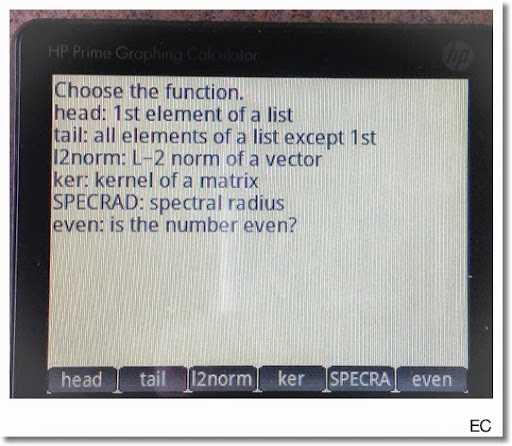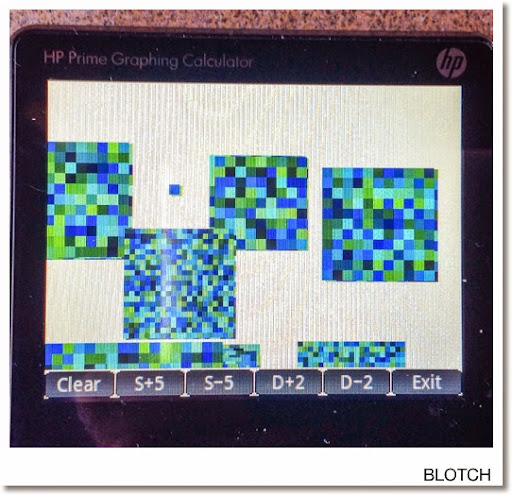## Sunday, April 20, 2014

### HP Prime: EC and BLOTCH - two programs using MOUSE and DRAWMENU

Happy Easter!
Happy 4/20 Day!
Happy Sunday!

These two programs for the HP Prime illustrate the use of DRAWMENU and MOUSE.

EC

Functions featured:
head: 1st element of a list
tail: all elements of a list except the 1st
l2norm: L-2 norm of a vector
ker: kernel of a matrix
even: is the number even?

Input: EC(argument)

The argument needs to be appropriate type of what you want to do.

For head and tail: the argument needs to be a list.
For l2norm: the argument needs to be a vector.
For ker and SPECRAD: the argument needs to be matrix
For even: we need an integer

Examples:
EC({7,8,9}), choosing head will return {7} while tail returns {8,9}.
EC([7,2,6,9]) while choosing l2norm returns √170.
EC([[1,4],[-3,-12]]) while choosing ker returns [[4, -1]].
EC([[1, 4],[-3, -12]]) while choosing SPECRAD returns 13.0384048104 (approximately)
For even, a result of 1 indicates that the number is even and 0 if the number is odd.

Program:

EXPORT EC(x)
BEGIN
// EWS 2014-04-20

LOCAL m,m1,mx,my;
WHILE MOUSE(1)≥0 DO END;
RECT;
TEXTOUT_P("Choose the function.",1,1,4);
TEXTOUT_P("head: 1st element of a list",1,18,4);
TEXTOUT_P("tail: all elements of a list except the 1st",1,35,4);
TEXTOUT_P("l2norm: L-2 norm of a vector",1,52,4);
TEXTOUT_P("ker: kernel of a matrix",1,69,4);
TEXTOUT_P("even: is the number even?",1,103,4);

REPEAT
m:=MOUSE;
m1:=m(1);
UNTIL SIZE(m1)>0;
mx:=m1(1);
my:=m1(2);

IF my≥220 AND my≤239 THEN

IF mx≥0 AND mx≤51 THEN
RETURN SUB(x,1,1);
END;

IF mx≥53 AND mx≤104 THEN
RETURN SUB(x,2,SIZE(x));
END;

IF mx≥106 AND mx≤157 THEN
RETURN exact(ABS(x));
END;

IF mx≥159 AND mx≤210 THEN
RETURN ker(x);
END;

IF mx≥212 AND mx≤263 THEN
RETURN CAS.SPECNORM(x);
END;

IF mx≥265 AND mx≤319 THEN
RETURN even(x);
END;

END;

END;BLOTCH

Blotch Drawing Program

S = size of the square blotch
D = size of each box in the blotch. Each box is a randomized color.

To draw a square blotch, just touch the screen outside the menu.

Input: BLOTCH( )

Program:

EXPORT BLOTCH( )
BEGIN
// EWS 04-20-2014

// Initialize
LOCAL m,m1,mx,my,j,k,r;
WHILE MOUSE(1)≥0 DO END;

// Clear Canvas
RECT;
LOCAL s:=50, d:=4;

// Menu - to be redrawn

// Start main loop
REPEAT

// Get mouse data
REPEAT
m:=MOUSE; m1:=m(1);
UNTIL SIZE(m1)>0;
mx:=m1(1); my:=m1(2);

// Clear Screen
IF (my≥220 AND my≤319) AND (mx≥0 AND mx≤51) THEN
RECT;
END;

// Change Size
IF (my≥220 AND my≤319) AND (mx≥53 AND mx≤104) THEN
IF s<80 THEN s:=s+5; END;
END;

IF (my≥220 AND my≤319) AND (mx≥106 AND mx≤157) THEN
IF s>5 THEN s:=s-5; END;
END;

// Change Depth
IF (my≥220 AND my≤319) AND (mx≥159 AND mx≤210) THEN
IF d<8 THEN d:=d+2; END;
END;

IF (my≥220 AND my≤319) AND (mx≥212 AND mx≤263) THEN
IF d>2 THEN d:=d-2; END;
END;

// Exit Key
IF (my≥220 AND my≤319) AND (mx≥256 AND mx≤319) THEN
BREAK;
END;

// Draw Blotch
FOR j FROM mx-s/2 TO mx+s/2 STEP d DO
FOR k FROM my-s/2 TO my+s/2 STEP d DO
r:=RANDINT(1677215);
RECT_P(j,k,j+d-1,k+d-1,r);
END; END;

// Close main loop
UNTIL (my≥220 AND my≤319) AND (mx≥256 AND mx≤319);

RECT_P(0,220,319,239);
TEXTOUT_P("DONE!",146,220,4,#FF0000h);
WAIT(-1);
END;As always, thank you for your comments, compliments, and questions. Happy Sunday everyone!

I will be heading to Seattle later this week - hope to blog from the Original Starbucks when I am there.

This blog is property of Edward Shore. 2014

### Texas Instruments: TI-36X Pro and TI-30X Pro Mathprint

Essentially, the Texas Instruments TI-36X Pro and the TI-30X MathPrint are functionally equivalent.  What makes the two calculators differe...